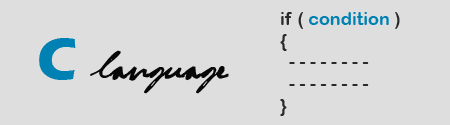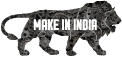Decision Control Statements .
Monday, July 27, 2009

# Decision Control Statements .

In this post my friend explains about Decision Control Statements in C Language. In programming languages if statement is very useful for decision making.Prasadh Teppala
Embedded Engineer,
IndiaIn program languages if statement is very useful for decision making.

In ‘C’ language the general format for an if statement is:

if ( condition )
{

Simple statement;
Simple statement;
…………….…..;
…………….…..;

}
Here sample statements will execute if and only if condition is true.

Condition Means

1) Normally condition are made with the Relational operators and Logic operators
Relational Operators: > , >= , <, <= , ==, !=.
Logic Operators: &&, || , !

Eg:
if ( i operator j )
One Statement:

Or

If ( i operator j )
{
More statements;
}

Here operator means Relational Operators and Logic Operators.
Here i and j integers, float, characters. Here characters compared by using their ASCII values.

2) Condition is also any number.

Eg:
int a; /* declaration of a */
If( a )
{
a = 10;
}

If we assign ‘0’ to the ‘a’ at the time of declaration , what is the value of the ‘a’ at the end of if block?
Ans: 0 only. 0 is always false.

If we assign ‘-1’ to the ‘a’ at the time of declaration, what is the value of the ‘a’ at the end of if block?
Ans: 10 only. Why Because -1 is true. Any Number other than 0 is always true. The number may be float, double or any.

3) Condition also assignment

Eg:
int a = 5; /* declaration of a */
If( a = 0 )
{
a = 10;
}

Or

int a = 5; /* declaration of a */
a = 0;
If( a )
{
a = 10;
}
Here what is the value of ‘a’? 5 or 0 or 10.
Ans: 0.
Initially ‘a’ was assigned with the 5.
Then in if statement ‘a’ assigned to 0. Therefore value of ‘a’ is 0. Now in place of condition 0 is there. According to the above point No: 2, 0 is always false, therefore the condition is false. The statements inside the ‘if’ block will not execute. There the value of ‘a’ is 0.

4) Condition containing expressions

Eg:
int a = 4; /* declaration and initialization of a */
int b = 2; /* declaration and initialization of b */
If( a/b = = 2 )
{
a = 10;
}
Here first execute the expression a/b which results 2 then it will compare. Therefore ‘a’ value after ‘if’ block is 10. The expressions are of any type and any side of the operator.

Part 2: Decision Control Statements - Part 2